Horn Driver Horn Fullrange Mids Coaxial speaker Woofer no longer available

## Faital

Since its inception in 1958 Faital has specialized in the design and manufacture of loudspeakers for a variety of industries.

.. Starting in the early 60’s with 40% of the total Italian market demand for loudspeakers, Faital steadily grew to become today one of Europe’s largest loudspeaker manufacturers.

.. All the while Faital also focused on improving and increasing its industrialization and technological capabilities.

.. Today Faital can count on 8 facilities worldwide and 18 automated production lines all equipped with custom quality check systems.

We are proud to be able to sell their products!

## Wooferopen a bigger photo

recommended cabinet 1:
closed cabinet with 1 L volume
from 206/130 Hz (-3dB/-8dB)

recommended cabinet 2:
3,7 L volume bass reflex cabinet
with HP50 reflex tube, 10 cm long.
from 103/83 Hz (-3dB/-8dB).

Faital Pro W6N8 120 A       order no. ah-FPW6N8120A       EUR 129,00excl. VAT: € 108.40 / \$ 120.33
17 cm midrange driver with paper cone. The magnet system is based on Neodymium technology.
• power handling (continuous/programme) = 120/240 W
• frequency range = 100-10000 Hz
• resonance frequency fs = 100 Hz
• impedance R = 8 Ohm
• DC resistance Re = 5,9 Ohm
• force factor BL = 10,3 N/A
• voice coil inductance L = 0,35 mH
• effective piston radiating area Sd = 113 cm2
• effective mechanical mass incl. air load mms = 11,7 g
• equivalent volume of compliance Vas = 3,9 l
• total Q factor Qts = 0,34 (Qms=6,8, Qes=0,41)
• voice coil diameter = 37 mm
• maximum peak linear excursion vibration xlin = +/- 5 mm
• mounting diameter d = 147 mm
• overall diameter d = 186,5 mm
• mounting depth (not countersunk) t = 88,5 mm
• mass m = 1,3 kg
PDF data sheet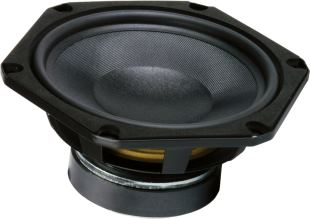open a bigger photo

recommended cabinet 1:
closed cabinet with 10,1 L volume
from 90/57 Hz (-3dB/-8dB)

recommended cabinet 2:
41 L volume bass reflex cabinet
with HP70 reflex tube, 7 cm long.
from 40/35 Hz (-3dB/-8dB).

Faital Pro 6 FE 100 A       order no. ah-FP6FE100A       EUR 49,00excl. VAT: € 41.18 / \$ 45.71
16 cm bass-midrange driver with high quality cone.
• power handling (continuous/programme) = 100/200 W
• frequency range = 63-5000 Hz
• resonance frequency fs = 61 Hz
• impedance R = 8 Ohm
• DC resistance Re = 5,4 Ohm
• force factor BL = 6,8 N/A
• voice coil inductance L = 0,5 mH
• effective piston radiating area Sd = 143 cm2
• effective mechanical mass incl. air load mms = 14 g
• equivalent volume of compliance Vas = 14,1 l
• total Q factor Qts = 0,55 (Qms=6, Qes=0,6)
• voice coil diameter = 32 mm
• maximum peak linear excursion vibration xlin = +/- 5,25 mm
• mounting diameter d = 147 mm
• overall diameter d = 181,2 mm
• mounting depth (not countersunk) t = 84,5 mm
• mass m = 1,3 kg
PDF data sheetopen a bigger photo

Faital Pro 6 FE 200 A       order no. ah-FP6FE200A       EUR 51,00excl. VAT: € 42.86 / \$ 47.57
15 cm midrange driver with paper cone.
• power handling (continuous/programme) = 130/260 W
• frequency range = 85-6000 Hz
• resonance frequency fs = 120 Hz
• impedance R = 8 Ohm
• DC resistance Re = 5,9 Ohm
• force factor BL = 8,2 N/A
• voice coil inductance L = 0,4 mH
• effective piston radiating area Sd = 118 cm2
• effective mechanical mass incl. air load mms = 11,5 g
• equivalent volume of compliance Vas = 3,03 l
• total Q factor Qts = 0,67 (Qms=6,23, Qes=0,75)
• voice coil diameter = 37 mm
• maximum peak linear excursion vibration xlin = +/- 4,67 mm
• mounting diameter d = 144 mm
• overall diameter d = 167,4 mm
• mounting depth (not countersunk) t = 77 mm
• mass m = 2 kg
PDF data sheet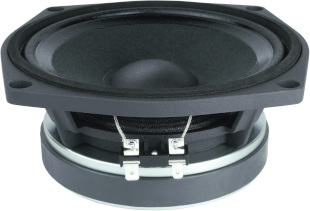open a bigger photo

recommended cabinet 1:
closed cabinet with 3,1 L volume
from 153/96 Hz (-3dB/-8dB)

recommended cabinet 2:
12,8 L volume bass reflex cabinet
with HP70 reflex tube, 8 cm long.
from 70/59 Hz (-3dB/-8dB).

Faital Pro 6 PR 110 A       order no. ah-FP6PR110A       EUR 93,00excl. VAT: € 78.15 / \$ 86.75
17 cm midrange driver with paper cone.
• power handling (continuous/programme) = 150/300 W
• frequency range = 100-6000 Hz
• resonance frequency fs = 100 Hz
• impedance R = 8 Ohm
• DC resistance Re = 6 Ohm
• force factor BL = 8,5 N/A
• voice coil inductance L = 0,22 mH
• effective piston radiating area Sd = 129 cm2
• effective mechanical mass incl. air load mms = 12 g
• equivalent volume of compliance Vas = 5 l
• total Q factor Qts = 0,53 (Qms=4,2, Qes=0,6)
• voice coil diameter = 37 mm
• maximum peak linear excursion vibration xlin = +/- 2,75 mm
• mounting diameter d = 147 mm
• overall diameter d = 186,5 mm
• mounting depth (not countersunk) t = 77,5 mm
• mass m = 2,2 kg
PDF data sheetopen a bigger photo

recommended cabinet 1:
closed cabinet with 0,81 L volume
from 235/149 Hz (-3dB/-8dB)

recommended cabinet 2:
2,7 L volume bass reflex cabinet
with HP50 reflex tube, 11 cm long.
from 124/97 Hz (-3dB/-8dB).

Faital Pro 6 PR 150 A       order no. ah-FP6PR150A       EUR 137,80excl. VAT: € 115.80 / \$ 128.54
17 cm midrange driver with paper cone.
• power handling (continuous/programme) = 150/300 W
• frequency range = 100-5000 Hz
• resonance frequency fs = 100 Hz
• impedance R = 8 Ohm
• DC resistance Re = 5,5 Ohm
• force factor BL = 11,6 N/A
• voice coil inductance L = 0,28 mH
• effective piston radiating area Sd = 129 cm2
• effective mechanical mass incl. air load mms = 13,5 g
• equivalent volume of compliance Vas = 4,4 l
• total Q factor Qts = 0,34 (Qms=8,8, Qes=0,35)
• voice coil diameter = 52 mm
• maximum peak linear excursion vibration xlin = +/- 2,65 mm
• mounting diameter d = 147 mm
• overall diameter d = 186,5 mm
• mounting depth (not countersunk) t = 89 mm
• mass m = 1,4 kg
PDF data sheet

## 8 inch wooferopen a bigger photo

Faital Pro 8 FE 200 A       order no. ah-FP8FE200A       EUR 55,00excl. VAT: € 46.22 / \$ 51.30
19 cm bass driver with paper cone.
• power handling (continuous/programme) = 130/260 W
• frequency range = 60-5000 Hz
• resonance frequency fs = 80 Hz
• impedance R = 8 Ohm
• DC resistance Re = 5,9 Ohm
• force factor BL = 8,2 N/A
• voice coil inductance L = 0,44 mH
• effective piston radiating area Sd = 191 cm2
• effective mechanical mass incl. air load mms = 15 g
• equivalent volume of compliance Vas = 13,6 l
• total Q factor Qts = 0,63 (Qms=13, Qes=0,66)
• voice coil diameter = 37 mm
• maximum peak linear excursion vibration xlin = +/- 4,67 mm
• mounting diameter d = 178 mm
• overall diameter d = 209,2 mm
• mounting depth (not countersunk) t = 89 mm
• mass m = 2,15 kg
PDF data sheetopen a bigger photo

recommended cabinet 1:
closed cabinet with 3,4 L volume
from 126/79 Hz (-3dB/-8dB)

recommended cabinet 2:
12 L volume bass reflex cabinet
with HP70 reflex tube, 16 cm long.
from 64/51 Hz (-3dB/-8dB).

Faital Pro 8 PR 200 A       order no. ah-FP8PR200A       EUR 199,80excl. VAT: € 167.90 / \$ 186.37
21 cm bass driver with paper cone. The magnet system is based on Neodymium technology.
• power handling (continuous/programme) = 200/400 W
• frequency range = 70-5000 Hz
• resonance frequency fs = 58 Hz
• impedance R = 8 Ohm
• DC resistance Re = 5,1 Ohm
• force factor BL = 11,5 N/A
• voice coil inductance L = 0,55 mH
• effective piston radiating area Sd = 196 cm2
• effective mechanical mass incl. air load mms = 27,2 g
• equivalent volume of compliance Vas = 15,1 l
• total Q factor Qts = 0,37 (Qms=9,4, Qes=0,38)
• voice coil diameter = 52 mm
• maximum peak linear excursion vibration xlin = +/- 8,15 mm
• mounting diameter d = 183 mm
• overall diameter d = 223,75 mm
• mounting depth (not countersunk) t = 116,7 mm
• mass m = 2,1 kg
PDF data sheetopen a bigger photo

recommended cabinet 1:
closed cabinet with 7,9 L volume
from 116/73 Hz (-3dB/-8dB)

recommended cabinet 2:
32 L volume bass reflex cabinet
with HP100 reflex tube, 13 cm long.
from 53/45 Hz (-3dB/-8dB).

Faital Pro W8N8 150 A       order no. ah-FPW8N8150A       EUR 147,80excl. VAT: € 124.20 / \$ 137.86
21 cm bass-midrange driver with paper cone. The magnet system is based on Neodymium technology.
• power handling (continuous/programme) = 150/300 W
• frequency range = 80-6300 Hz
• resonance frequency fs = 76 Hz
• impedance R = 8 Ohm
• DC resistance Re = 5,8 Ohm
• force factor BL = 9,9 N/A
• voice coil inductance L = 0,55 mH
• effective piston radiating area Sd = 206 cm2
• effective mechanical mass incl. air load mms = 20,9 g
• equivalent volume of compliance Vas = 12,6 l
• total Q factor Qts = 0,52 (Qms=4,72, Qes=0,59)
• voice coil diameter = 52 mm
• maximum peak linear excursion vibration xlin = +/- 5,52 mm
• mounting diameter d = 183 mm
• overall diameter d = 223,75 mm
• mounting depth (not countersunk) t = 116,7 mm
• mass m = 1,7 kg
PDF data sheetopen a bigger photo

Faital Pro W8N8 200 A       order no. ah-FPW8N8200A       EUR 163,00excl. VAT: € 136.97 / \$ 152.04
21 cm bass-midrange driver with paper cone. The magnet system is based on Neodymium technology.
• power handling (continuous/programme) = 200/400 W
• frequency range = 80-5000 Hz
• resonance frequency fs = 74 Hz
• impedance R = 8 Ohm
• DC resistance Re = 5,1 Ohm
• force factor BL = 8,9 N/A
• voice coil inductance L = 0,6 mH
• effective piston radiating area Sd = 177 cm2
• effective mechanical mass incl. air load mms = 23 g
• equivalent volume of compliance Vas = 9 l
• total Q factor Qts = 0,61 (Qms=5,3, Qes=0,69)
• voice coil diameter = 52 mm
• maximum peak linear excursion vibration xlin = +/- 8,48 mm
• mounting diameter d = 183 mm
• overall diameter d = 223,75 mm
• mounting depth (not countersunk) t = 116,7 mm
• mass m = 1,8 kg
PDF data sheet

## 10 inch woofer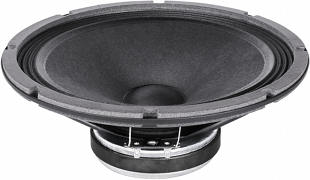open a bigger photo

Faital Pro 10 FE 200 A       order no. ah-FP10FE200A       EUR 57,80excl. VAT: € 48.57 / \$ 53.91
24 cm bass-midrange driver with paper cone.
• power handling (continuous/programme) = 150/300 W
• frequency range = 50-4000 Hz
• resonance frequency fs = 55 Hz
• impedance R = 8 Ohm
• DC resistance Re = 5,9 Ohm
• force factor BL = 8,2 N/A
• voice coil inductance L = 0,42 mH
• effective piston radiating area Sd = 332 cm2
• effective mechanical mass incl. air load mms = 24,5 g
• equivalent volume of compliance Vas = 53,4 l
• total Q factor Qts = 0,69 (Qms=11,3, Qes=0,74)
• voice coil diameter = 37 mm
• maximum peak linear excursion vibration xlin = +/- 4,67 mm
• mounting diameter d = 233,8 mm
• overall diameter d = 256,8 mm
• mounting depth (not countersunk) t = 102 mm
• mass m = 2,2 kg
PDF data sheetopen a bigger photo

recommended cabinet 1:
closed cabinet with 2,3 L volume
from 183/116 Hz (-3dB/-8dB)

recommended cabinet 2:
5,8 L volume bass reflex cabinet
with HP70 reflex tube, 16 cm long.
from 107/81 Hz (-3dB/-8dB).

Faital Pro 10 FH 500 A       order no. ah-FP10FH500A       EUR 225,00excl. VAT: € 189.08 / \$ 209.87
25 cm bass-midrange driver with paper cone. The magnet system is based on Neodymium technology.
• power handling (continuous/programme) = 500/1000 W
• frequency range = 60-4000 Hz
• resonance frequency fs = 60 Hz
• impedance R = 8 Ohm
• DC resistance Re = 5,1 Ohm
• force factor BL = 17,9 N/A
• voice coil inductance L = 0,85 mH
• effective piston radiating area Sd = 328 cm2
• effective mechanical mass incl. air load mms = 47 g
• equivalent volume of compliance Vas = 22,85 l
• total Q factor Qts = 0,26 (Qms=3,28, Qes=0,28)
• voice coil diameter = 77 mm
• maximum peak linear excursion vibration xlin = +/- 7,5 mm
• mounting diameter d = 232 mm
• overall diameter d = 261 mm
• mounting depth (not countersunk) t = 141,3 mm
• mass m = 3,4 kg
PDF data sheetopen a bigger photo

Faital Pro 10 FH 500 B       order no. ah-FP10FH500B       EUR 225,00excl. VAT: € 189.08 / \$ 209.87
25 cm bass-midrange driver with paper cone.
• power handling (continuous/programme) = 500/1000 W
• frequency range = 60-4000 Hz
• impedance R = 16 Ohm
• effective piston radiating area Sd = 328 cm2
• voice coil diameter = 77 mm
• maximum peak linear excursion vibration xlin = +/- 7,5 mm
• mounting diameter d = 232 mm
• overall diameter d = 261 mm
• mounting depth (not countersunk) t = 141,3 mm
• mass m = 3,4 kg
PDF data sheetopen a bigger photo

Faital Pro 10 FH 500 C       order no. ah-FP10FH500C       EUR 225,00excl. VAT: € 189.08 / \$ 209.87
25 cm bass-midrange driver with paper cone.
• power handling (continuous/programme) = 500/1000 W
• frequency range = 60-4000 Hz
• impedance R = 4 Ohm
• effective piston radiating area Sd = 328 cm2
• voice coil diameter = 77 mm
• maximum peak linear excursion vibration xlin = +/- 7,5 mm
• mounting diameter d = 232 mm
• overall diameter d = 261 mm
• mounting depth (not countersunk) t = 141,3 mm
• mass m = 3,4 kg
PDF data sheetopen a bigger photo

recommended cabinet 1:
closed cabinet with 2,6 L volume
from 165/104 Hz (-3dB/-8dB)

recommended cabinet 2:
7,4 L volume bass reflex cabinet
with HP70 reflex tube, 16 cm long.
from 92/71 Hz (-3dB/-8dB).

Faital Pro 10 FH 520 A       order no. ah-FP10FH520A       EUR 225,00excl. VAT: € 189.08 / \$ 209.87
25 cm bass-midrange driver with paper cone. The magnet system is based on Neodymium technology.
• power handling (continuous/programme) = 600/1200 W
• frequency range = 60-4000 Hz
• resonance frequency fs = 60 Hz
• impedance R = 8 Ohm
• DC resistance Re = 5,1 Ohm
• force factor BL = 18 N/A
• voice coil inductance L = 0,9 mH
• effective piston radiating area Sd = 328 cm2
• effective mechanical mass incl. air load mms = 50,3 g
• equivalent volume of compliance Vas = 20,45 l
• total Q factor Qts = 0,32 (Qms=11,1, Qes=0,3)
• voice coil diameter = 77 mm
• maximum peak linear excursion vibration xlin = +/- 7,25 mm
• mounting diameter d = 232 mm
• overall diameter d = 261 mm
• mounting depth (not countersunk) t = 141,3 mm
• mass m = 3,7 kg
PDF data sheetopen a bigger photo

recommended cabinet 1:
closed cabinet with 1,2 L volume
from 198/125 Hz (-3dB/-8dB)

recommended cabinet 2:
2,7 L volume bass reflex cabinet
with HP70 reflex tube, 35 cm long.
from 120/90 Hz (-3dB/-8dB).

Faital Pro 10 HP 1020 A       order no. ah-FP10HP1020A       EUR 339,80excl. VAT: € 285.55 / \$ 316.96
25 cm bass driver with paper cone. The magnet system is based on Neodymium technology.
• power handling (continuous/programme) = 700/1400 W
• frequency range = 60-2500 Hz
• resonance frequency fs = 60 Hz
• impedance R = 8 Ohm
• DC resistance Re = 5,5 Ohm
• force factor BL = 24,5 N/A
• voice coil inductance L = 0,85 mH
• effective piston radiating area Sd = 315 cm2
• effective mechanical mass incl. air load mms = 72,5 g
• equivalent volume of compliance Vas = 13,66 l
• total Q factor Qts = 0,23 (Qms=6,5, Qes=0,25)
• voice coil diameter = 100 mm
• maximum peak linear excursion vibration xlin = +/- 9 mm
• mounting diameter d = 232 mm
• overall diameter d = 261 mm
• mounting depth (not countersunk) t = 141,3 mm
• mass m = 5,2 kg
PDF data sheetopen a bigger photo

recommended cabinet 1:
closed cabinet with 4,3 L volume
from 170/108 Hz (-3dB/-8dB)

recommended cabinet 2:
11,9 L volume bass reflex cabinet
with HP100 reflex tube, 19 cm long.
from 97/74 Hz (-3dB/-8dB).

Faital Pro 10 PR 300 A       order no. ah-FP10PR300A       EUR 189,80excl. VAT: € 159.50 / \$ 177.04
25 cm bass-midrange driver with paper cone. The magnet system is based on Neodymium technology.
• power handling (continuous/programme) = 300/600 W
• frequency range = 60-5000 Hz
• resonance frequency fs = 60 Hz
• impedance R = 8 Ohm
• DC resistance Re = 5,4 Ohm
• force factor BL = 14,1 N/A
• voice coil inductance L = 0,6 mH
• effective piston radiating area Sd = 322 cm2
• effective mechanical mass incl. air load mms = 28,2 g
• equivalent volume of compliance Vas = 36,7 l
• total Q factor Qts = 0,26 (Qms=5,8, Qes=0,29)
• voice coil diameter = 65 mm
• maximum peak linear excursion vibration xlin = +/- 4,92 mm
• mounting diameter d = 232 mm
• overall diameter d = 261 mm
• mounting depth (not countersunk) t = 115,3 mm
• mass m = 2,3 kg
PDF data sheetopen a bigger photo

Faital Pro 10 PR 300 B       order no. ah-FP10PR300B       EUR 189,80excl. VAT: € 159.50 / \$ 177.04
25 cm bass-midrange driver with paper cone. The magnet system is based on Neodymium technology.
• power handling (continuous/programme) = 300/600 W
• frequency range = 60-5000 Hz
• impedance R = 16 Ohm
• effective piston radiating area Sd = 322 cm2
• voice coil diameter = 65 mm
• maximum peak linear excursion vibration xlin = +/- 4,9 mm
• mounting diameter d = 232 mm
• overall diameter d = 261 mm
• mounting depth (not countersunk) t = 115,3 mm
• mass m = 2,3 kg
PDF data sheetopen a bigger photo

recommended cabinet 1:
closed cabinet with 5,1 L volume
from 141/89 Hz (-3dB/-8dB)

recommended cabinet 2:
17 L volume bass reflex cabinet
with HP100 reflex tube, 19 cm long.
from 74/58 Hz (-3dB/-8dB).

Faital Pro 10 PR 310 A       order no. ah-FP10PR310A       EUR 157,80excl. VAT: € 132.61 / \$ 147.19
25 cm bass-midrange driver with paper cone.
• power handling (continuous/programme) = 300/600 W
• frequency range = 60-4000 Hz
• resonance frequency fs = 60 Hz
• impedance R = 8 Ohm
• DC resistance Re = 5,4 Ohm
• force factor BL = 14,4 N/A
• voice coil inductance L = 0,64 mH
• effective piston radiating area Sd = 322 cm2
• effective mechanical mass incl. air load mms = 36,9 g
• equivalent volume of compliance Vas = 28 l
• total Q factor Qts = 0,34 (Qms=5,7, Qes=0,36)
• voice coil diameter = 65 mm
• maximum peak linear excursion vibration xlin = +/- 4,92 mm
• mounting diameter d = 230 mm
• overall diameter d = 261 mm
• mounting depth (not countersunk) t = 109 mm
• mass m = 4,1 kg
PDF data sheet

## 12 inch wooferopen a bigger photo

recommended cabinet 1:
closed cabinet with 6,9 L volume
from 143/90 Hz (-3dB/-8dB)

recommended cabinet 2:
17 L volume bass reflex cabinet
with HP100 reflex tube, 20 cm long.
from 85/64 Hz (-3dB/-8dB).

Faital Pro 12 FH 500 A       order no. ah-FP12FH500A       EUR 261,80excl. VAT: € 220.00 / \$ 244.20
30 cm bass-midrange driver with paper cone. The magnet system is based on Neodymium technology.
• power handling (continuous/programme) = 500/1000 W
• frequency range = 45-4000 Hz
• resonance frequency fs = 45 Hz
• impedance R = 8 Ohm
• DC resistance Re = 5,1 Ohm
• force factor BL = 17,5 N/A
• voice coil inductance L = 0,83 mH
• effective piston radiating area Sd = 487 cm2
• effective mechanical mass incl. air load mms = 56 g
• equivalent volume of compliance Vas = 75,1 l
• total Q factor Qts = 0,25 (Qms=6,9, Qes=0,26)
• voice coil diameter = 77 mm
• maximum peak linear excursion vibration xlin = +/- 7,5 mm
• mounting diameter d = 284 mm
• overall diameter d = 316 mm
• mounting depth (not countersunk) t = 155,75 mm
• mass m = 3,9 kg
PDF data sheetopen a bigger photo

recommended cabinet 1:
closed cabinet with 5,9 L volume
from 148/94 Hz (-3dB/-8dB)

recommended cabinet 2:
13,8 L volume bass reflex cabinet
with HP100 reflex tube, 23 cm long.
from 90/67 Hz (-3dB/-8dB).

Faital Pro 12 FH 510 A       order no. ah-FP12FH510A       EUR 225,00excl. VAT: € 189.08 / \$ 209.87
30 cm bass-midrange driver with paper cone.
• power handling (continuous/programme) = 500/1000 W
• frequency range = 45-3150 Hz
• resonance frequency fs = 45 Hz
• impedance R = 8 Ohm
• DC resistance Re = 5,1 Ohm
• force factor BL = 18,9 N/A
• voice coil inductance L = 0,9 mH
• effective piston radiating area Sd = 489 cm2
• effective mechanical mass incl. air load mms = 61 g
• equivalent volume of compliance Vas = 69,56 l
• total Q factor Qts = 0,24 (Qms=10,9, Qes=0,25)
• voice coil diameter = 77 mm
• maximum peak linear excursion vibration xlin = +/- 7,5 mm
• mounting diameter d = 282 mm
• overall diameter d = 316 mm
• mounting depth (not countersunk) t = 140 mm
• mass m = 8 kg
PDF data sheet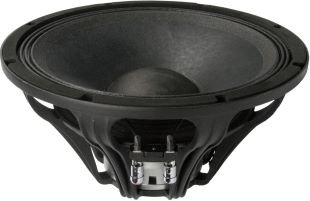open a bigger photo

recommended cabinet 1:
closed cabinet with 8,2 L volume
from 137/87 Hz (-3dB/-8dB)

recommended cabinet 2:
23 L volume bass reflex cabinet
with HP100 reflex tube, 14 cm long.
from 77/59 Hz (-3dB/-8dB).

Faital Pro 12 FH 520 A       order no. ah-FP12FH520A       EUR 295,80excl. VAT: € 248.57 / \$ 275.91
30 cm bass-midrange driver with paper cone. The magnet system is based on Neodymium technology.
• power handling (continuous/programme) = 600/1200 W
• frequency range = 50-4000 Hz
• resonance frequency fs = 50 Hz
• impedance R = 8 Ohm
• DC resistance Re = 5,1 Ohm
• force factor BL = 17,3 N/A
• voice coil inductance L = 0,74 mH
• effective piston radiating area Sd = 500 cm2
• effective mechanical mass incl. air load mms = 56 g
• equivalent volume of compliance Vas = 64,2 l
• total Q factor Qts = 0,28 (Qms=11,1, Qes=0,3)
• voice coil diameter = 77 mm
• maximum peak linear excursion vibration xlin = +/- 7,25 mm
• mounting diameter d = 284 mm
• overall diameter d = 316 mm
• mounting depth (not countersunk) t = 155,75 mm
• mass m = 4,2 kg
PDF data sheetopen a bigger photo

recommended cabinet 1:
closed cabinet with 9,2 L volume
from 106/67 Hz (-3dB/-8dB)

recommended cabinet 2:
30 L volume bass reflex cabinet
with HP100 reflex tube, 19 cm long.
from 56/44 Hz (-3dB/-8dB).

Faital Pro 12 HP 1010 A       order no. ah-FP12HP1010A       EUR 269,80excl. VAT: € 226.72 / \$ 251.66
30 cm bass driver with paper cone.
• power handling (continuous/programme) = 700/1400 W
• frequency range = 45-3150 Hz
• resonance frequency fs = 45 Hz
• impedance R = 8 Ohm
• DC resistance Re = 5,5 Ohm
• force factor BL = 19,8 N/A
• voice coil inductance L = 1,33 mH
• effective piston radiating area Sd = 496 cm2
• effective mechanical mass incl. air load mms = 87,2 g
• equivalent volume of compliance Vas = 50 l
• total Q factor Qts = 0,34 (Qms=9,4, Qes=0,35)
• voice coil diameter = 100 mm
• maximum peak linear excursion vibration xlin = +/- 9,25 mm
• mounting diameter d = 282 mm
• overall diameter d = 316 mm
• mounting depth (not countersunk) t = 142 mm
• mass m = 9,22 kg
PDF data sheet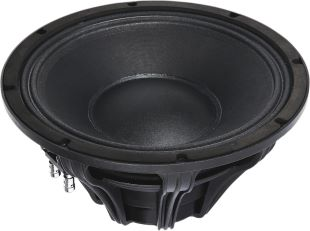open a bigger photo

recommended cabinet 1:
closed cabinet with 3,7 L volume
from 162/102 Hz (-3dB/-8dB)

recommended cabinet 2:
9,7 L volume bass reflex cabinet
with HP100 reflex tube, 29 cm long.
from 93/71 Hz (-3dB/-8dB).

Faital Pro 12 HP 1020 A       order no. ah-FP12HP1020A       EUR 371,80excl. VAT: € 312.44 / \$ 346.81
30 cm bass driver with paper cone. The magnet system is based on Neodymium technology.
• power handling (continuous/programme) = 700/1400 W
• frequency range = 55-3150 Hz
• resonance frequency fs = 55 Hz
• impedance R = 8 Ohm
• DC resistance Re = 5,5 Ohm
• force factor BL = 24,3 N/A
• voice coil inductance L = 1,1 mH
• effective piston radiating area Sd = 494 cm2
• effective mechanical mass incl. air load mms = 86 g
• equivalent volume of compliance Vas = 33,7 l
• total Q factor Qts = 0,27 (Qms=9,4, Qes=0,28)
• voice coil diameter = 100 mm
• maximum peak linear excursion vibration xlin = +/- 9 mm
• mounting diameter d = 284 mm
• overall diameter d = 316 mm
• mounting depth (not countersunk) t = 155,75 mm
• mass m = 5,8 kg
PDF data sheetopen a bigger photo

recommended cabinet 1:
closed cabinet with 4,1 L volume
from 120/75 Hz (-3dB/-8dB)

recommended cabinet 2:
12,1 L volume bass reflex cabinet
with HP100 reflex tube, 45 cm long.
from 66/51 Hz (-3dB/-8dB).

Faital Pro 12 HP 1030 A       order no. ah-FP12HP1030A       EUR 329,80excl. VAT: € 277.14 / \$ 307.63
30 cm bass driver with paper cone.
• power handling (continuous/programme) = 1000/2000 W
• frequency range = 45-1600 Hz
• resonance frequency fs = 45 Hz
• impedance R = 8 Ohm
• DC resistance Re = 5 Ohm
• force factor BL = 24,3 N/A
• voice coil inductance L = 1,35 mH
• effective piston radiating area Sd = 469 cm2
• effective mechanical mass incl. air load mms = 130,5 g
• equivalent volume of compliance Vas = 29,91 l
• total Q factor Qts = 0,3 (Qms=13,75, Qes=0,31)
• voice coil diameter = 100 mm
• maximum peak linear excursion vibration xlin = +/- 12,45 mm
• mounting diameter d = 282 mm
• overall diameter d = 316 mm
• mounting depth (not countersunk) t = 147 mm
• mass m = 11,1 kg
PDF data sheet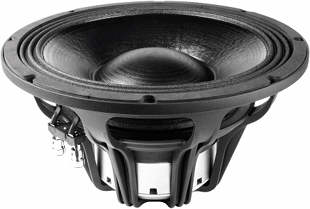open a bigger photo

recommended cabinet 1:
closed cabinet with 3,6 L volume
from 128/81 Hz (-3dB/-8dB)

recommended cabinet 2:
10 L volume bass reflex cabinet
with HP100 reflex tube, 48 cm long.
from 73/55 Hz (-3dB/-8dB).

Faital Pro 12 HP 1060 A       order no. ah-FP12HP1060A       EUR 437,00excl. VAT: € 367.23 / \$ 407.62
30 cm bass driver with paper cone.
• power handling (continuous/programme) = 1000/2000 W
• frequency range = 45-2500 Hz
• resonance frequency fs = 45 Hz
• impedance R = 8 Ohm
• DC resistance Re = 5 Ohm
• force factor BL = 24,8 N/A
• voice coil inductance L = 1,38 mH
• effective piston radiating area Sd = 469 cm2
• effective mechanical mass incl. air load mms = 126,9 g
• equivalent volume of compliance Vas = 30,8 l
• total Q factor Qts = 0,28 (Qms=12,1, Qes=0,29)
• voice coil diameter = 100 mm
• maximum peak linear excursion vibration xlin = +/- 12,45 mm
• mounting diameter d = 282 mm
• overall diameter d = 316 mm
• mounting depth (not countersunk) t = 168,75 mm
• mass m = 6,6 kg
PDF data sheetopen a bigger photo

recommended cabinet 1:
closed cabinet with 17 L volume
from 111/70 Hz (-3dB/-8dB)

recommended cabinet 2:
58 L volume bass reflex cabinet
with 2x HP100 reflex tube, 14 cm long.
from 57/45 Hz (-3dB/-8dB).

Faital Pro 12 PR 300 A       order no. ah-FP12PR300A       EUR 205,00excl. VAT: € 172.27 / \$ 191.22
30 cm bass-midrange driver with paper cone. The magnet system is based on Neodymium technology.
• power handling (continuous/programme) = 300/600 W
• frequency range = 50-5000 Hz
• resonance frequency fs = 50 Hz
• impedance R = 8 Ohm
• DC resistance Re = 5,4 Ohm
• force factor BL = 14,1 N/A
• voice coil inductance L = 0,42 mH
• effective piston radiating area Sd = 489 cm2
• effective mechanical mass incl. air load mms = 43,4 g
• equivalent volume of compliance Vas = 79,2 l
• total Q factor Qts = 0,39 (Qms=9,92, Qes=0,37)
• voice coil diameter = 65 mm
• maximum peak linear excursion vibration xlin = +/- 4,92 mm
• mounting diameter d = 283 mm
• overall diameter d = 315,2 mm
• mounting depth (not countersunk) t = 140,6 mm
• mass m = 2,5 kg
PDF data sheet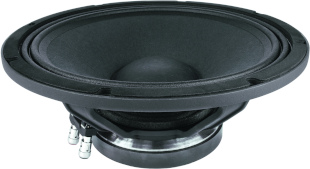open a bigger photo

recommended cabinet 1:
closed cabinet with 17 L volume
from 109/68 Hz (-3dB/-8dB)

recommended cabinet 2:
65 L volume bass reflex cabinet
with 2x HP100 reflex tube, 12 cm long.
from 54/43 Hz (-3dB/-8dB).

Faital Pro 12 PR 310 A       order no. ah-FP12PR310A       EUR 178,00excl. VAT: € 149.58 / \$ 166.03
30 cm bass-midrange driver with paper cone.
• power handling (continuous/programme) = 300/600 W
• frequency range = 50-4000 Hz
• resonance frequency fs = 54 Hz
• impedance R = 8 Ohm
• DC resistance Re = 5,4 Ohm
• force factor BL = 14,4 N/A
• voice coil inductance L = 0,61 mH
• effective piston radiating area Sd = 489 cm2
• effective mechanical mass incl. air load mms = 46,9 g
• equivalent volume of compliance Vas = 62,83 l
• total Q factor Qts = 0,4 (Qms=11,6, Qes=0,41)
• voice coil diameter = 65 mm
• maximum peak linear excursion vibration xlin = +/- 4,92 mm
• mounting diameter d = 282 mm
• overall diameter d = 316 mm
• mounting depth (not countersunk) t = 135 mm
• mass m = 4,3 kg
PDF data sheet

## 15 inch wooferopen a bigger photo

recommended cabinet 1:
closed cabinet with 27 L volume
from 90/57 Hz (-3dB/-8dB)

recommended cabinet 2:
81 L volume bass reflex cabinet
with 2x HP100 reflex tube, 16 cm long.
from 49/38 Hz (-3dB/-8dB).

Faital Pro 15 FH 500 A       order no. ah-FP15FH500A       EUR 297,80excl. VAT: € 250.25 / \$ 277.78
38 cm bass-midrange driver with paper cone. The magnet system is based on Neodymium technology.
• power handling (continuous/programme) = 500/1000 W
• frequency range = 35-3150 Hz
• resonance frequency fs = 35 Hz
• impedance R = 8 Ohm
• DC resistance Re = 5,1 Ohm
• force factor BL = 19,2 N/A
• voice coil inductance L = 1,3 mH
• effective piston radiating area Sd = 800 cm2
• effective mechanical mass incl. air load mms = 104 g
• equivalent volume of compliance Vas = 180,5 l
• total Q factor Qts = 0,31 (Qms=10,4, Qes=0,32)
• voice coil diameter = 77 mm
• maximum peak linear excursion vibration xlin = +/- 9,25 mm
• mounting diameter d = 356 mm
• overall diameter d = 393 mm
• mounting depth (not countersunk) t = 181,3 mm
• mass m = 4,3 kg
PDF data sheetopen a bigger photo

recommended cabinet 1:
closed cabinet with 17 L volume
from 107/67 Hz (-3dB/-8dB)

recommended cabinet 2:
44 L volume bass reflex cabinet
with 2x HP100 reflex tube, 24 cm long.
from 63/47 Hz (-3dB/-8dB).

Faital Pro 15 FH 510 A       order no. ah-FP15FH510A       EUR 258,00excl. VAT: € 216.81 / \$ 240.66
38 cm bass-midrange driver with paper cone.
• power handling (continuous/programme) = 500/1000 W
• frequency range = 35-3150 Hz
• resonance frequency fs = 35 Hz
• impedance R = 8 Ohm
• DC resistance Re = 5,1 Ohm
• force factor BL = 21 N/A
• voice coil inductance L = 1,4 mH
• effective piston radiating area Sd = 800 cm2
• effective mechanical mass incl. air load mms = 108 g
• equivalent volume of compliance Vas = 173,8 l
• total Q factor Qts = 0,26 (Qms=10,7, Qes=0,27)
• voice coil diameter = 77 mm
• maximum peak linear excursion vibration xlin = +/- 9,25 mm
• mounting diameter d = 354 mm
• overall diameter d = 393 mm
• mounting depth (not countersunk) t = 180 mm
• mass m = 8,4 kg
PDF data sheetopen a bigger photo

recommended cabinet 1:
closed cabinet with 43 L volume
from 76/48 Hz (-3dB/-8dB)

recommended cabinet 2:
161 L volume bass reflex cabinet
with 2x HP100 reflex tube, 8 cm long.
from 38/31 Hz (-3dB/-8dB).

Faital Pro 15 FH 520 A       order no. ah-FP15FH520A       EUR 333,00excl. VAT: € 279.83 / \$ 310.61
38 cm bass-midrange driver with paper cone. The magnet system is based on Neodymium technology.
• power handling (continuous/programme) = 600/1200 W
• frequency range = 40-4000 Hz
• resonance frequency fs = 38 Hz
• impedance R = 8 Ohm
• DC resistance Re = 5,6 Ohm
• force factor BL = 18,4 N/A
• voice coil inductance L = 0,89 mH
• effective piston radiating area Sd = 813 cm2
• effective mechanical mass incl. air load mms = 105 g
• equivalent volume of compliance Vas = 156,6 l
• total Q factor Qts = 0,28 (Qms=10,1, Qes=0,41)
• voice coil diameter = 77 mm
• maximum peak linear excursion vibration xlin = +/- 8,75 mm
• mounting diameter d = 356 mm
• overall diameter d = 393 mm
• mounting depth (not countersunk) t = 181,3 mm
• mass m = 4,6 kg
PDF data sheet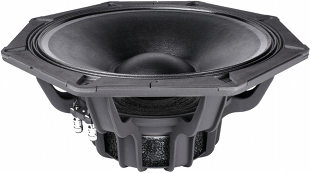open a bigger photo

recommended cabinet 1:
closed cabinet with 20 L volume
from 101/64 Hz (-3dB/-8dB)

recommended cabinet 2:
58 L volume bass reflex cabinet
with 2x HP100 reflex tube, 19 cm long.
from 56/43 Hz (-3dB/-8dB).

Faital Pro 15 FX 560 A       order no. ah-FP15FX560A       EUR 379,80excl. VAT: € 319.16 / \$ 354.27
39 cm bass driver with paper cone. The magnet system is based on Neodymium technology.
• power handling (continuous/programme) = 700/1400 W
• frequency range = 40-4000 Hz
• resonance frequency fs = 38 Hz
• impedance R = 8 Ohm
• DC resistance Re = 5,2 Ohm
• force factor BL = 21,5 N/A
• voice coil inductance L = 0,64 mH
• effective piston radiating area Sd = 813 cm2
• effective mechanical mass incl. air load mms = 115 g
• equivalent volume of compliance Vas = 143 l
• total Q factor Qts = 0,3 (Qms=6,76, Qes=0,31)
• voice coil diameter = 77 mm
• maximum peak linear excursion vibration xlin = +/- 9,42 mm
• mounting diameter d = 354 mm
• overall diameter d = 418,4 mm
• mounting depth (not countersunk) t = 185 mm
• mass m = 7,5 kg
PDF data sheetopen a bigger photo

recommended cabinet 1:
closed cabinet with 21 L volume
from 87/55 Hz (-3dB/-8dB)

recommended cabinet 2:
74 L volume bass reflex cabinet
with 2x HP100 reflex tube, 20 cm long.
from 44/35 Hz (-3dB/-8dB).

Faital Pro 15 HP 1010 A       order no. ah-FP15HP1010A       EUR 291,80excl. VAT: € 245.21 / \$ 272.18
38 cm bass driver with paper cone.
• power handling (continuous/programme) = 700/1400 W
• frequency range = 40-2500 Hz
• resonance frequency fs = 40 Hz
• impedance R = 8 Ohm
• DC resistance Re = 5,3 Ohm
• force factor BL = 22 N/A
• voice coil inductance L = 1,8 mH
• effective piston radiating area Sd = 759 cm2
• effective mechanical mass incl. air load mms = 138,9 g
• equivalent volume of compliance Vas = 93,14 l
• total Q factor Qts = 0,37 (Qms=10, Qes=0,38)
• voice coil diameter = 100 mm
• maximum peak linear excursion vibration xlin = +/- 9,75 mm
• mounting diameter d = 354 mm
• overall diameter d = 393 mm
• mounting depth (not countersunk) t = 183 mm
• mass m = 9,75 kg
PDF data sheet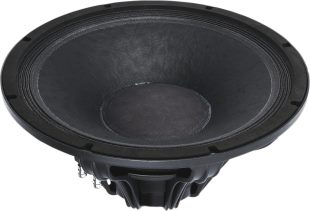open a bigger photo

recommended cabinet 1:
closed cabinet with 12 L volume
from 118/74 Hz (-3dB/-8dB)

recommended cabinet 2:
32 L volume bass reflex cabinet
with 2x HP100 reflex tube, 29 cm long.
from 68/51 Hz (-3dB/-8dB).

Faital Pro 15 HP 1020 A       order no. ah-FP15HP1020A       EUR 399,00excl. VAT: € 335.29 / \$ 372.18
38 cm bass driver with paper cone. The magnet system is based on Neodymium technology.
• power handling (continuous/programme) = 700/1400 W
• frequency range = 40-4000 Hz
• resonance frequency fs = 40 Hz
• impedance R = 8 Ohm
• DC resistance Re = 5,5 Ohm
• force factor BL = 24,2 N/A
• voice coil inductance L = 1,2 mH
• effective piston radiating area Sd = 759 cm2
• effective mechanical mass incl. air load mms = 118 g
• equivalent volume of compliance Vas = 109,6 l
• total Q factor Qts = 0,27 (Qms=10,2, Qes=0,28)
• voice coil diameter = 100 mm
• maximum peak linear excursion vibration xlin = +/- 9 mm
• mounting diameter d = 356 mm
• overall diameter d = 393 mm
• mounting depth (not countersunk) t = 181,3 mm
• mass m = 6,15 kg
PDF data sheetopen a bigger photo

recommended cabinet 1:
closed cabinet with 15 L volume
from 94/59 Hz (-3dB/-8dB)

recommended cabinet 2:
50 L volume bass reflex cabinet
with 2x HP100 reflex tube, 28 cm long.
from 50/39 Hz (-3dB/-8dB).

Faital Pro 15 HP 1030 A       order no. ah-FP15HP1030A       EUR 379,80excl. VAT: € 319.16 / \$ 354.27
38 cm bass driver with paper cone.
• power handling (continuous/programme) = 1000/2000 W
• frequency range = 40-2000 Hz
• resonance frequency fs = 40 Hz
• impedance R = 8 Ohm
• DC resistance Re = 5 Ohm
• force factor BL = 24,3 N/A
• voice coil inductance L = 1,4 mH
• effective piston radiating area Sd = 780 cm2
• effective mechanical mass incl. air load mms = 165 g
• equivalent volume of compliance Vas = 82,8 l
• total Q factor Qts = 0,34 (Qms=11,83, Qes=0,35)
• voice coil diameter = 100 mm
• maximum peak linear excursion vibration xlin = +/- 12,45 mm
• mounting diameter d = 354 mm
• overall diameter d = 393 mm
• mounting depth (not countersunk) t = 188 mm
• mass m = 11,7 kg
PDF data sheetopen a bigger photo

recommended cabinet 1:
closed cabinet with 13 L volume
from 103/65 Hz (-3dB/-8dB)

recommended cabinet 2:
39 L volume bass reflex cabinet
with 2x HP100 reflex tube, 31 cm long.
from 56/43 Hz (-3dB/-8dB).

Faital Pro 15 HP 1060 A       order no. ah-FP15HP1060A       EUR 479,80excl. VAT: € 403.19 / \$ 447.54
38 cm bass driver with paper cone.
• power handling (continuous/programme) = 1000/2000 W
• frequency range = 40-2000 Hz
• resonance frequency fs = 40 Hz
• impedance R = 8 Ohm
• DC resistance Re = 5 Ohm
• force factor BL = 24,8 N/A
• voice coil inductance L = 1,32 mH
• effective piston radiating area Sd = 780 cm2
• effective mechanical mass incl. air load mms = 156,3 g
• equivalent volume of compliance Vas = 87,5 l
• total Q factor Qts = 0,31 (Qms=11,4, Qes=0,32)
• voice coil diameter = 100 mm
• maximum peak linear excursion vibration xlin = +/- 12,45 mm
• mounting diameter d = 354 mm
• overall diameter d = 393 mm
• mounting depth (not countersunk) t = 194,3 mm
• mass m = 7,1 kg
PDF data sheetopen a bigger photo

Faital Pro 15 HP 1060 C       order no. ah-FP15HP1060C       EUR 479,80excl. VAT: € 403.19 / \$ 447.54
38 cm bass driver with paper cone.
• power handling (continuous/programme) = 1000/2000 W
• frequency range = 40-2000 Hz
• impedance R = 4 Ohm
• effective piston radiating area Sd = 780 cm2
• voice coil diameter = 100 mm
• maximum peak linear excursion vibration xlin = +/- 12,5 mm
• mounting diameter d = 354 mm
• overall diameter d = 393 mm
• mounting depth (not countersunk) t = 194,3 mm
• mass m = 7,1 kg
PDF data sheetopen a bigger photo

recommended cabinet 1:
closed cabinet with 36 L volume
from 87/55 Hz (-3dB/-8dB)

recommended cabinet 2:
111 L volume bass reflex cabinet
with 2x HP100 reflex tube, 10 cm long.
from 47/36 Hz (-3dB/-8dB).

Faital Pro 15 PR 400 A       order no. ah-FP15PR400A       EUR 267,80excl. VAT: € 225.04 / \$ 249.80
38 cm bass driver with paper cone. The magnet system is based on Neodymium technology.
• power handling (continuous/programme) = 400/800 W
• frequency range = 35-4000 Hz
• resonance frequency fs = 35 Hz
• impedance R = 8 Ohm
• DC resistance Re = 5,1 Ohm
• force factor BL = 16,7 N/A
• voice coil inductance L = 0,75 mH
• effective piston radiating area Sd = 805,5 cm2
• effective mechanical mass incl. air load mms = 85,2 g
• equivalent volume of compliance Vas = 223 l
• total Q factor Qts = 0,32 (Qms=6,02, Qes=0,34)
• voice coil diameter = 77 mm
• maximum peak linear excursion vibration xlin = +/- 5,75 mm
• mounting diameter d = 356 mm
• overall diameter d = 393 mm
• mounting depth (not countersunk) t = 165 mm
• mass m = 3,6 kg
PDF data sheet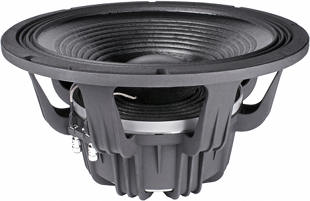open a bigger photo

recommended cabinet 1:
closed cabinet with 13,7 L volume
from 92/58 Hz (-3dB/-8dB)

recommended cabinet 2:
44 L volume bass reflex cabinet
with 2x HP100 reflex tube, 35 cm long.
from 49/38 Hz (-3dB/-8dB).

Faital Pro 15 XL 1400 A       order no. ah-FP15XL1400A       EUR 585,80excl. VAT: € 492.27 / \$ 546.42
38 cm bass driver with paper cone. The magnet system is based on Neodymium technology.
• power handling (continuous/programme) = 1400/2800 W
• frequency range = 40-2500 Hz
• resonance frequency fs = 38 Hz
• impedance R = 8 Ohm
• DC resistance Re = 5,4 Ohm
• force factor BL = 26,7 N/A
• voice coil inductance L = 1,6 mH
• effective piston radiating area Sd = 780 cm2
• effective mechanical mass incl. air load mms = 190 g
• equivalent volume of compliance Vas = 79,7 l
• total Q factor Qts = 0,33 (Qms=10,4, Qes=0,34)
• voice coil diameter = 100 mm
• maximum peak linear excursion vibration xlin = +/- 12,9 mm
• mounting diameter d = 354 mm
• overall diameter d = 393 mm
• mounting depth (not countersunk) t = 217 mm
• mass m = 11,75 kg
PDF data sheet

## 18 inch wooferopen a bigger photo

recommended cabinet 1:
closed cabinet with 74 L volume
from 69/43 Hz (-3dB/-8dB)

recommended cabinet 2:
250 L volume bass reflex cabinet
with 4x HP100 reflex tube, 19 cm long.
from 36/28 Hz (-3dB/-8dB).

Faital Pro 18 FH 500 A       order no. ah-FP18FH500A       EUR 339,00excl. VAT: € 284.87 / \$ 316.21
44 cm bass driver with paper cone. The magnet system is based on Neodymium technology.
• power handling (continuous/programme) = 600/1200 W
• frequency range = 30-3150 Hz
• resonance frequency fs = 30 Hz
• impedance R = 8 Ohm
• DC resistance Re = 5,1 Ohm
• force factor BL = 19 N/A
• voice coil inductance L = 1,35 mH
• effective piston radiating area Sd = 1134 cm2
• effective mechanical mass incl. air load mms = 137 g
• equivalent volume of compliance Vas = 374,8 l
• total Q factor Qts = 0,35 (Qms=12,5, Qes=0,36)
• voice coil diameter = 77 mm
• maximum peak linear excursion vibration xlin = +/- 9,25 mm
• mounting diameter d = 424 mm
• overall diameter d = 460 mm
• mounting depth (not countersunk) t = 202 mm
• mass m = 4,6 kg
PDF data sheetopen a bigger photo

recommended cabinet 1:
closed cabinet with 47 L volume
from 82/52 Hz (-3dB/-8dB)

recommended cabinet 2:
134 L volume bass reflex cabinet
with 4x HP100 reflex tube, 29 cm long.
from 46/35 Hz (-3dB/-8dB).

Faital Pro 18 FH 510 A       order no. ah-FP18FH510A       EUR 269,80excl. VAT: € 226.72 / \$ 251.66
44 cm bass driver with paper cone.
• power handling (continuous/programme) = 600/1200 W
• frequency range = 30-2500 Hz
• resonance frequency fs = 30 Hz
• impedance R = 8 Ohm
• DC resistance Re = 5,1 Ohm
• force factor BL = 21 N/A
• voice coil inductance L = 1,06 mH
• effective piston radiating area Sd = 1134 cm2
• effective mechanical mass incl. air load mms = 139 g
• equivalent volume of compliance Vas = 369,4 l
• total Q factor Qts = 0,29 (Qms=13,6, Qes=0,3)
• voice coil diameter = 77 mm
• maximum peak linear excursion vibration xlin = +/- 9,25 mm
• mounting diameter d = 421 mm
• overall diameter d = 460 mm
• mounting depth (not countersunk) t = 201,5 mm
• mass m = 8,75 kg
PDF data sheetopen a bigger photo

recommended cabinet 1:
closed cabinet with 59 L volume
from 70/44 Hz (-3dB/-8dB)

recommended cabinet 2:
221 L volume bass reflex cabinet
with 4x HP100 reflex tube, 21 cm long.
from 35/28 Hz (-3dB/-8dB).

Faital Pro 18 HP 1010 A       order no. ah-FP18HP1010A       EUR 313,80excl. VAT: € 263.70 / \$ 292.70
44 cm bass driver with paper cone.
• power handling (continuous/programme) = 1000/2000 W
• frequency range = 35-2000 Hz
• resonance frequency fs = 35 Hz
• impedance R = 8 Ohm
• DC resistance Re = 5,3 Ohm
• force factor BL = 22 N/A
• voice coil inductance L = 1,8 mH
• effective piston radiating area Sd = 1134 cm2
• effective mechanical mass incl. air load mms = 175,2 g
• equivalent volume of compliance Vas = 215,3 l
• total Q factor Qts = 0,4 (Qms=7,8, Qes=0,42)
• voice coil diameter = 100 mm
• maximum peak linear excursion vibration xlin = +/- 9,75 mm
• mounting diameter d = 421 mm
• overall diameter d = 460 mm
• mounting depth (not countersunk) t = 203,5 mm
• mass m = 10,1 kg
PDF data sheetopen a bigger photo

recommended cabinet 1:
closed cabinet with 23 L volume
from 103/65 Hz (-3dB/-8dB)

recommended cabinet 2:
60 L volume bass reflex cabinet
with 2x HP100 reflex tube, 17 cm long.
from 59/45 Hz (-3dB/-8dB).

Faital Pro 18 HP 1020 A       order no. ah-FP18HP1020A       EUR 479,80excl. VAT: € 403.19 / \$ 447.54
44 cm bass driver with paper cone. The magnet system is based on Neodymium technology.
• power handling (continuous/programme) = 1000/2000 W
• frequency range = 35-1600 Hz
• resonance frequency fs = 35 Hz
• impedance R = 8 Ohm
• DC resistance Re = 5,4 Ohm
• force factor BL = 28 N/A
• voice coil inductance L = 1,7 mH
• effective piston radiating area Sd = 1134 cm2
• effective mechanical mass incl. air load mms = 182 g
• equivalent volume of compliance Vas = 207,2 l
• total Q factor Qts = 0,27 (Qms=9,74, Qes=0,28)
• voice coil diameter = 100 mm
• maximum peak linear excursion vibration xlin = +/- 9,5 mm
• mounting diameter d = 424 mm
• overall diameter d = 460 mm
• mounting depth (not countersunk) t = 202 mm
• mass m = 6,45 kg
PDF data sheetopen a bigger photo

recommended cabinet 1:
closed cabinet with 51 L volume
from 67/42 Hz (-3dB/-8dB)

recommended cabinet 2:
195 L volume bass reflex cabinet
with 4x HP100 reflex tube, 30 cm long.
from 33/27 Hz (-3dB/-8dB).

Faital Pro 18 HP 1030 A       order no. ah-FP18HP1030A       EUR 418,00excl. VAT: € 351.26 / \$ 389.90
44 cm bass driver with paper cone.
• power handling (continuous/programme) = 1200/2400 W
• frequency range = 35-1600 Hz
• resonance frequency fs = 35 Hz
• impedance R = 8 Ohm
• DC resistance Re = 5 Ohm
• force factor BL = 24,3 N/A
• voice coil inductance L = 1,48 mH
• effective piston radiating area Sd = 1124 cm2
• effective mechanical mass incl. air load mms = 228,6 g
• equivalent volume of compliance Vas = 162,1 l
• total Q factor Qts = 0,41 (Qms=11,6, Qes=0,43)
• voice coil diameter = 100 mm
• maximum peak linear excursion vibration xlin = +/- 12,45 mm
• mounting diameter d = 421 mm
• overall diameter d = 460 mm
• mounting depth (not countersunk) t = 208,5 mm
• mass m = 12,1 kg
PDF data sheetopen a bigger photo

recommended cabinet 1:
closed cabinet with 36 L volume
from 89/56 Hz (-3dB/-8dB)

recommended cabinet 2:
119 L volume bass reflex cabinet
with 4x HP100 reflex tube, 27 cm long.
from 47/37 Hz (-3dB/-8dB).

Faital Pro 18 HP 1040 A       order no. ah-FP18HP1040A       EUR 495,80excl. VAT: € 416.64 / \$ 462.47
44 cm bass driver with paper cone. The magnet system is based on Neodymium technology.
• power handling (continuous/programme) = 1000/2000 W
• frequency range = 38-2500 Hz
• resonance frequency fs = 38 Hz
• impedance R = 8 Ohm
• DC resistance Re = 5,5 Ohm
• force factor BL = 24,5 N/A
• voice coil inductance L = 1,36 mH
• effective piston radiating area Sd = 1134 cm2
• effective mechanical mass incl. air load mms = 163 g
• equivalent volume of compliance Vas = 196,3 l
• total Q factor Qts = 0,34 (Qms=8,75, Qes=0,35)
• voice coil diameter = 100 mm
• maximum peak linear excursion vibration xlin = +/- 9 mm
• mounting diameter d = 424 mm
• overall diameter d = 460 mm
• mounting depth (not countersunk) t = 202 mm
• mass m = 6,45 kg
PDF data sheetopen a bigger photo

recommended cabinet 1:
closed cabinet with 39 L volume
from 76/48 Hz (-3dB/-8dB)

recommended cabinet 2:
140 L volume bass reflex cabinet
with 4x HP100 reflex tube, 34 cm long.
from 39/31 Hz (-3dB/-8dB).

Faital Pro 18 HP 1060 A       order no. ah-FP18HP1060A       EUR 537,00excl. VAT: € 451.26 / \$ 500.90
44 cm bass driver with paper cone. The magnet system is based on Neodymium technology.
• power handling (continuous/programme) = 1200/2400 W
• frequency range = 35-1600 Hz
• resonance frequency fs = 35 Hz
• impedance R = 8 Ohm
• DC resistance Re = 5 Ohm
• force factor BL = 24,8 N/A
• voice coil inductance L = 1,26 mH
• effective piston radiating area Sd = 1124 cm2
• effective mechanical mass incl. air load mms = 211,8 g
• equivalent volume of compliance Vas = 175 l
• total Q factor Qts = 0,37 (Qms=11,1, Qes=0,38)
• voice coil diameter = 100 mm
• maximum peak linear excursion vibration xlin = +/- 12,45 mm
• mounting diameter d = 422 mm
• overall diameter d = 460 mm
• mounting depth (not countersunk) t = 215 mm
• mass m = 7,3 kg
PDF data sheetopen a bigger photo

recommended cabinet 1:
closed cabinet with 39 L volume
from 71/45 Hz (-3dB/-8dB)

recommended cabinet 2:
135 L volume bass reflex cabinet
with 4x HP100 reflex tube, 43 cm long.
from 37/29 Hz (-3dB/-8dB).

Faital Pro 18 XL 1600 A       order no. ah-FP18XL1600A       EUR 635,80excl. VAT: € 534.29 / \$ 593.06
44 cm bass driver with paper cone. The magnet system is based on Neodymium technology.
• power handling (continuous/programme) = 1600/3200 W
• frequency range = 30-1500 Hz
• resonance frequency fs = 32 Hz
• impedance R = 8 Ohm
• DC resistance Re = 5,4 Ohm
• force factor BL = 26,7 N/A
• voice coil inductance L = 1,45 mH
• effective piston radiating area Sd = 1124 cm2
• effective mechanical mass incl. air load mms = 240 g
• equivalent volume of compliance Vas = 184,7 l
• total Q factor Qts = 0,36 (Qms=9,3, Qes=0,37)
• voice coil diameter = 100 mm
• maximum peak linear excursion vibration xlin = +/- 12,9 mm
• mounting diameter d = 422 mm
• overall diameter d = 460 mm
• mounting depth (not countersunk) t = 231 mm
• mass m = 12,2 kg
PDF data sheet
 Horn Driver Horn Fullrange Mids Coaxial speaker Woofer no longer available
The cabinet volume calculations are executed considering an external resistance of 0.3 Ohm. We are using, if no other data is known or available, the information supplied by the manufacturer.

Please note: the frequency response doesn't say much about the cut-off frequency inside the cabinet! Therefore, we mostly give you 2 examples with dimensioning considerations and an indication, how low the loudspeaker will reproduce.

Home